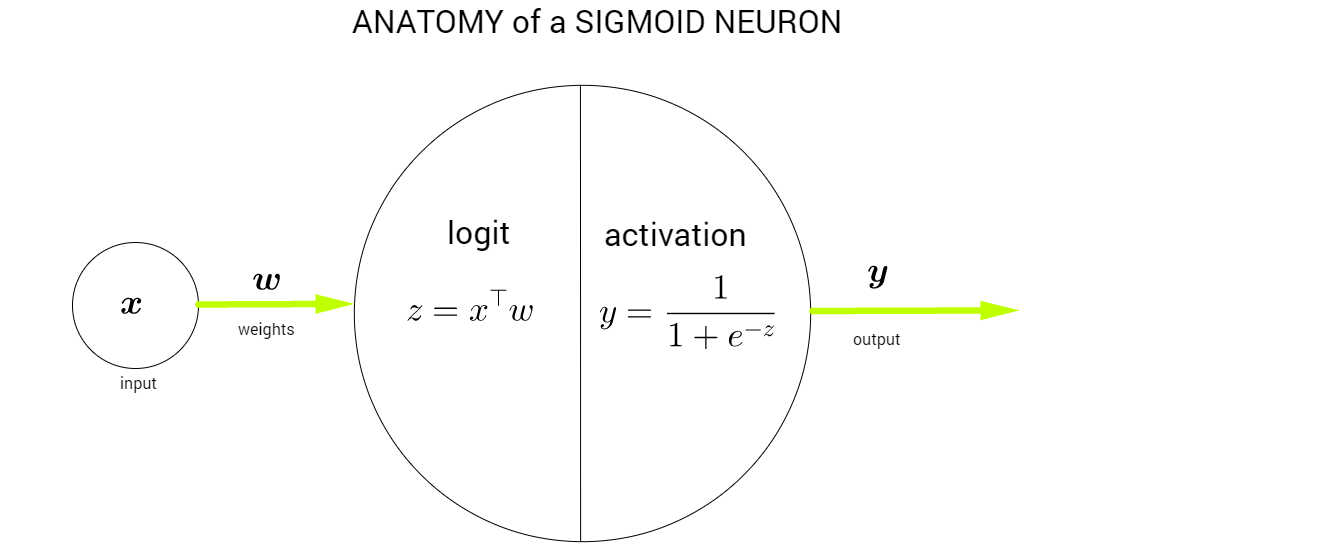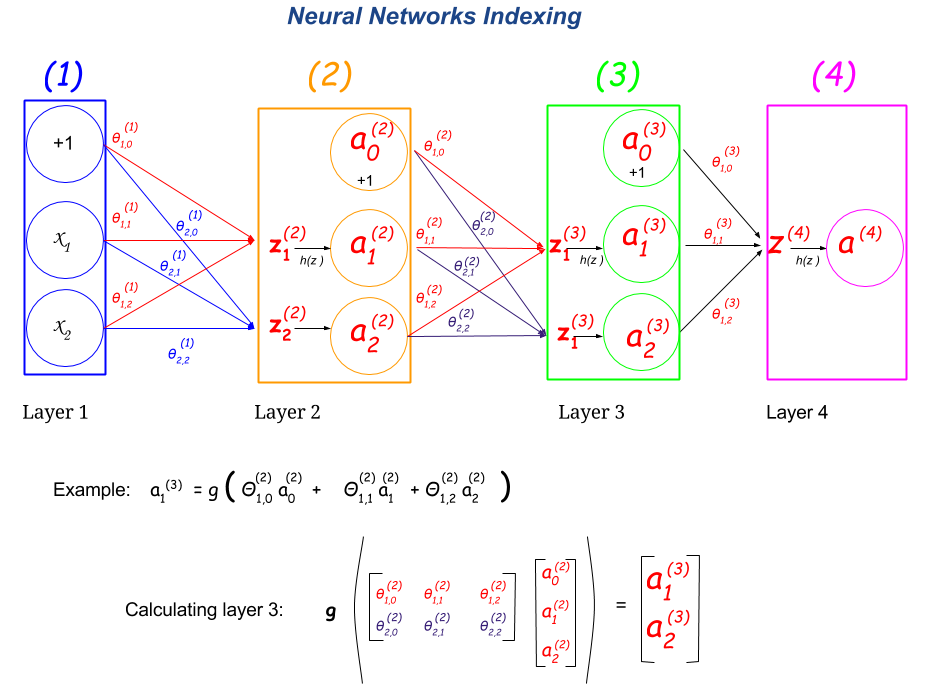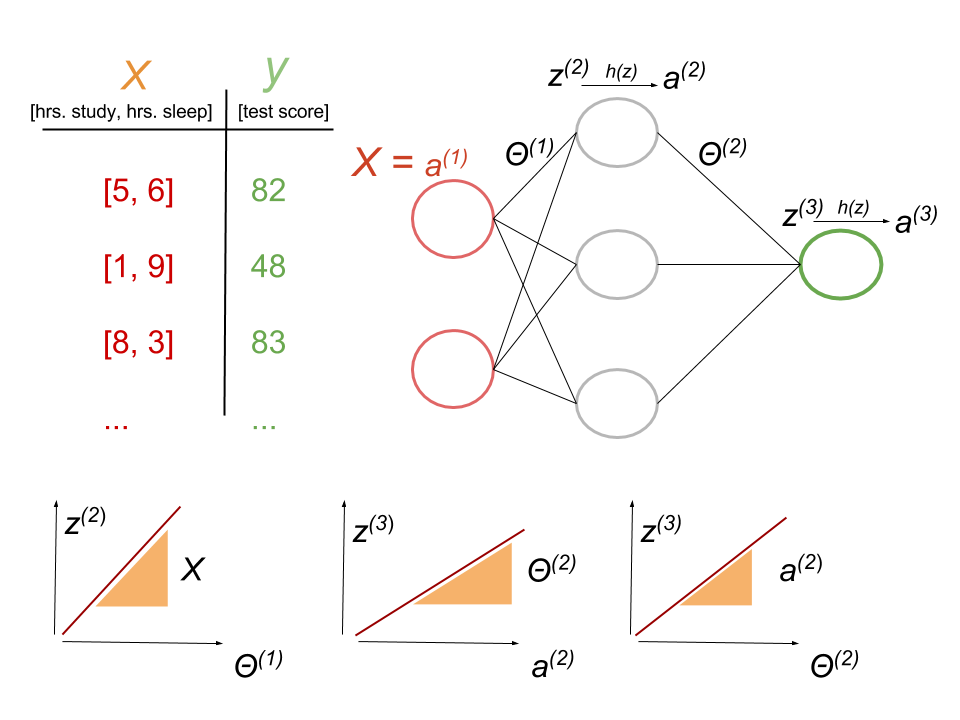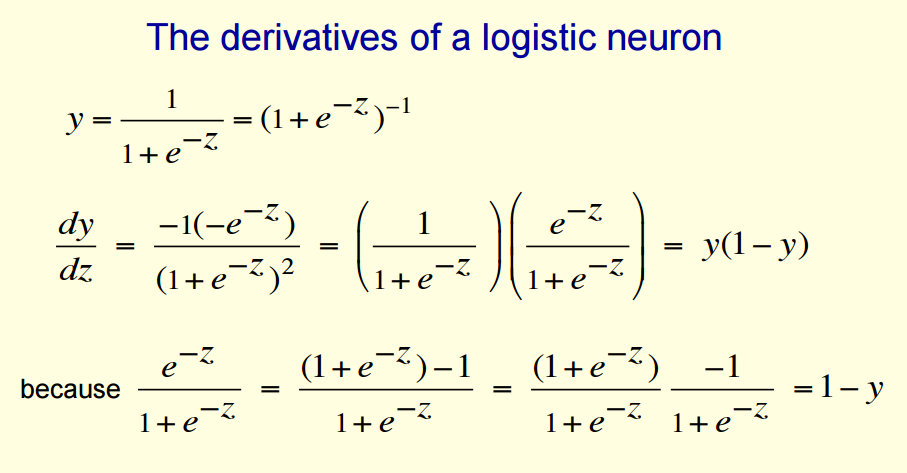### NEURAL NETWORKS:

The anatomy of a neural network is as follows:The index notation of a neural network is as follows:Notice that $$\large x_1 =a_1^{(1)}$$ and that $$\large x_2 = a_2^{(1)}.$$

$$L$$ is the total number of layers. $$s_l$$ is the number of units (excluding the bias unit) in layer $$l$$.

##### Example with regression after this youtube video:$$a_j^{(l)}$$ is the activation node $$j$$ in layer $$l$$.

We need to calculate $$\large \frac {\partial J}{\partial \Theta}$$. The weights $$\large \Theta$$ are spread in two matrices: $$\large \Theta^{1}$$ and $$\large \Theta^{2}$$:

$$\color{red}{\large \Theta^{1}=\begin{bmatrix}\theta_{11}^{(1)} & \theta_{12}^{(1)} & \theta_{13}^{(1)}\\\theta_{21}^{(1)} & \theta_{22}^{(1)} & \theta_{23}^{(1)} \end{bmatrix}}$$ and $$\color{green}{\large \Theta^{2}=\begin{bmatrix}\theta_{11}^{(2)} \\ \theta_{21}^{(2)} \\ \theta_{31}^{(2)} \end{bmatrix}}$$

We need to calculate:

$$\color{red}{\large \frac{\partial J}{\partial \Theta^{(1)}} = \begin{bmatrix}\frac{\partial J}{\partial \theta_{11}^{(1)}} & \frac{\partial J}{\partial \theta_{12}^{(1)}} & \frac{\partial J}{\partial \theta_{13}^{(1)}}\\ \frac{\partial J}{\partial \theta_{21}^{(1)}} & \frac{\partial J}{\partial \theta_{22}^{(1)}} & \frac{\partial J}{\partial \theta_{23}^{(1)}}\end{bmatrix}}$$ and $$\color{green}{\large \frac {\partial J}{\partial \Theta^{(2)}}=\begin{bmatrix}\frac{\partial J}{\partial\theta_{11}^{(2)}} \\ \frac{\partial J}{\partial\theta_{21}^{(2)}} \\ \frac{\partial J}{\partial\theta_{31}^{(2)}} \end{bmatrix}}$$

The cost function adds cost from each example. In what follows we will use the cost function of a regression model as $$\frac{1}{2}\sum (y-\hat y)^2$$, although it would be more accurate as $$\frac{1}{2m}\sum (y-\hat y)^2$$.

$$\large \color{green}{\frac{\partial J}{\partial \Theta^{(2)}}}=\frac{\partial \sum \frac{1}{2}(y-\hat y)^2}{\partial \Theta^{(2)}}=\sum \frac{\partial \frac{1}{2}(y-\hat y)^2}{\partial \Theta^{(2)}}$$ by he sum rule in differentiation that says that $$\frac{d}{dx}(u+v)=\frac{du}{dx}+\frac{dv}{dx}$$

Focusing on the expression inside the sum, and applying the chain rule, $$\large(f\circ g)'=(f'\circ g)\cdot g'$$ or $$\large\frac{dz}{dx}=\frac{dz}{dy}\cdot\frac{dy}{dx}$$.

$$\large \color{green}{\frac{\partial J}{\partial \Theta^{(2)}}}=\frac{\partial J}{\partial y}\frac{\partial y}{\partial \Theta^{(2)}}=\frac{\partial \left(\frac{1}{2}\sum (y-\hat y)^2\right)}{\partial y}\frac{\partial y}{\partial \Theta^{(2)}}=\color{fuchsia}{(y-\hat y)}\cdot \frac{\color{fuchsia}{-}\partial \hat y}{\partial \Theta^{(2)}}$$

Since $$\hat y$$ is the sigmoid activation function of $$z^{(3)}$$, which is $$g\big(z^{(3)}\big)$$ we can apply the chain rule again:

$$\large \color{green}{\frac{\partial J}{\partial \Theta^{(2)}}}=(y-\hat y)\cdot \frac{-\partial \hat y}{\partial z^{(3)}}\cdot \frac{\partial z^{(3)}}{\partial \Theta^{(2)}}=\color{fuchsia}{-(y-\hat y)}\cdot \color{blue}{g'(z^{(3)})} \cdot \color{lime}{\frac{\partial z^{(3)}}{\partial \Theta^{(2)}}}\tag 1$$

The derivative of the sigmoid activation function with respect to $$z$$ is:

$$\large \color{blue}{g'(z)}=\frac{e^{-z}}{\left(1+e^{-z}\right)^2}=\left(\frac{1}{1+e^{-z}}\right)\times \left(1 - \frac{1}{1+e^{-z}}\right)=\color{blue}{\hat y\left(1-\hat y\right)}$$ (see embedded mathy picture below for derivation).

Now, $$\large z^{(3)}=a^{(2)}\Theta^{(2)}$$, and hence $$a$$ is the slope of $$z$$ with respect to $$z$$ for each synapse. Therefore $$\large \color{lime}{\frac{\partial z^{(3)}}{\partial \Theta^{(2)}}=\begin{bmatrix}a_{11}^{(2)}&a_{12}^{(2)}&a_{13}^{(2)}\\a_{21}^{(2)}&a_{22}^{(2)}&a_{23}^{(2)}\\a_{31}^{(2)}&a_{32}^{(2)}&a_{33}^{(2)}\end{bmatrix}}$$

$$\large \color{green}{\frac{\partial J}{\partial \Theta^{(2)}}}=\color{fuchsia}{-(y-\hat y)}\cdot \color{blue}{\left (\hat y\,(1-\hat y)\right)}\cdot \color{lime}{a^{(2)}}\tag{*}$$

In Hinton’s Coursera course on NN, this expression (*) is seen as:

$\frac{\partial E}{\partial w_i}=\sum_n \frac{\partial y^n}{\partial w_i}\color{orange}{\frac{\partial E}{\partial y^n}}=\color{orange}{-}\sum_n \color{red}{x_i^n}\,\color{blue}{y^n \,(1-y^n)}\,\color{orange}{(t^n-y^n)}$

where $$E$$ stands for the error, $$w_i$$ is the weight $$i$$, $$n$$ is the index of the examples in the training set, and $$t$$ stands for training case.

because,

$\frac{\partial y^n}{\partial w_i}=\color{red}{\frac{\partial z}{\partial w_i}}\color{blue}{\frac{dy}{dz}}=\color{red}{x_i^n}\,\color{blue}{y^n(1-y^n)}$

the latter part in blue is derived here:and because

$E=\frac{1}{2}\displaystyle\sum_{n\in\text{ex's train set}}(t^n-y^n)^2$

and the derivative with respect to $$y^n$$ is:

$\color{orange}{\frac{\partial E}{\partial y^n}=-(t^n-y^n)}.$

Going back to Eq. 1: